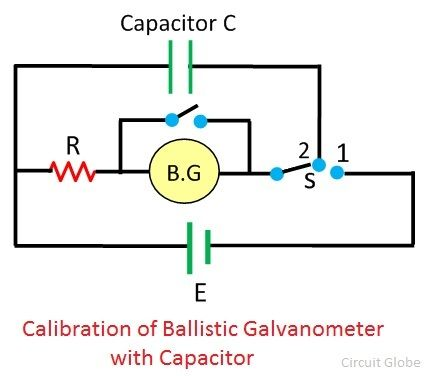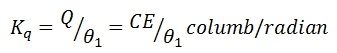# Ballistic Galvanometer

Definition: The galvanometer which is used for estimating the quantity of charge flow through it is called the ballistic galvanometer. The working principle of the ballistic galvanometer is very simple. It depends on the deflection of the coil which is directly proportional to the charge passes through it. The galvanometer measures the majority of the charge passes through it in spite of current.

## Construction of Ballistic Galvanometer

The ballistic galvanometer consists coil of copper wire which is wound on the non-conducting frame of the galvanometer. The phosphorous bronze suspends the coil between the north and south poles of a magnet. For increasing the magnetic flux the iron core places within the coil. The lower portion of the coil connects with the spring. This spring provides the restoring torque to the coil.When the charge passes through the galvanometer, their coil starts moving and gets an impulse. The impulse of the coil is proportional to the charges passes through it. The actual reading of the galvanometer achieves by using the coil having a high moment of inertia. The moment of inertia means the body oppose the angular movement. If the coil has a high moment of inertia, then their oscillations are large. Thus, accurate reading is obtained.

### Theory of Ballistic Galvanometer

Consider the rectangular coil having N number of turns placed in a uniform magnetic field. Let l be the length and b be the breadth of the coil. The area of the coil is given asWhen the current passes through the coil, the torque acts on it. The given expression determines the magnitude of the torque.Let the current flow through the coil for very short duration says dt and it is expressed asIf the current passing through the coil for t seconds, the expression becomesThe q be the total charge passes through the coil. The moment of inertia of the coil is given by l, and the angular velocity through ω. The expression gives the angular momentum of the coilThe angular momentum of the coil is equal to the force acting on the coil. Thus from equation (4) and (5), we get.The Kinetic Energy (K) deflects the coil through an angle θ, and this deflection is restored through the spring.The resorting torque of the coil is equal to their deflection. Thus,The periodic oscillation of the coil is given asBy multiplying the equation (7) from the above equation we getOn substituting the value of equation (6) in the equation (8) we getThe K is the constant of the ballistic galvanometer.

### Calibration of Galvanometer

The calibration of the galvanometer is the process of determining its constant value by the help of the practical experiments. The following are the methods used for determining the constant of the ballistic galvanometer.

### Using a Capacitor

The charging and discharging of the capacitor gives the values of the ballistic galvanometer constant. The circuit arrangement for the calibration of a ballistic galvanometer using the capacitor is shown in the figure below.The circuit uses two pole switch S and the unknown EMF source E. When the switch S connects to terminal 2 then the capacitor becomes charged. Similarly, when the switch connects to terminal 1, then the capacitor becomes discharges through the resistor R, connected in series with the ballistic galvanometer.

The discharge current of the capacitor deflects the coil of ballistic galvanometer through an angle θ. The formula calculates constant of the galvanometer### Using a Mutual Inductance

The constant of the ballistic galvanometer determines through the mutual inductance between the coils. The arrangement of the ballistic galvanometer requires two coils; primary and secondary. The primary coil is energised by knowing voltage source.Because of the mutual induction, the current induces in the secondary circuit. And this current is used for the calibration of the ballistic galvanometer.